# PyTorch 13：nn 网络层：池化层、线性层和激活函数层

## PyTorch 学习笔记

Posted by YEY on December 16, 2020

# Lecture 13 nn 网络层：池化层、全连接层和激活函数层

## 1. 池化层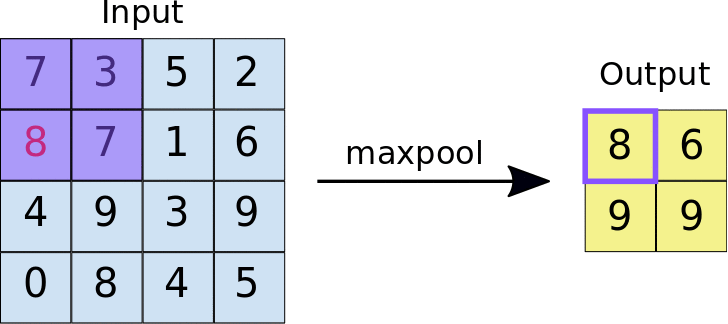• “收集”：多变少。
• “总结”：最大值/平均值。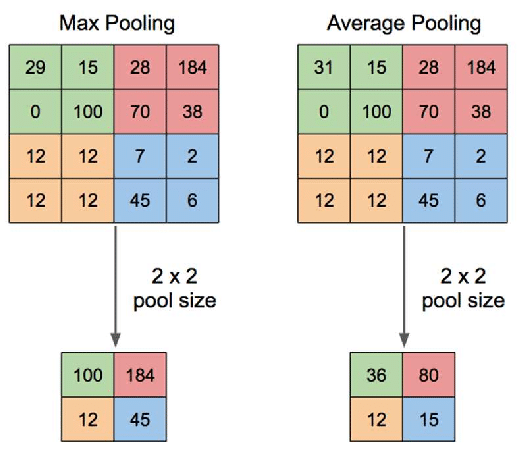#### nn.MaxPool2d

1
2
3
4
5
6
7
8
nn.MaxPool2d(
kernel_size,
stride=None,
dilation=1,
return_indices=False,
ceil_mode=False
)


• kernel_size：池化核尺寸。
• stride：步长。
• padding：填充个数。
• dilation：池化核间隔大小。
• ceil_mode：尺寸是否向上取整。用于计算输出特征图尺寸，默认设置为向下取整。
• return_indices：记录池化像素索引。通常在最大值反池化上采样时使用。

1
2
3
4
5
6
7
8
9
10
11
12
13
14
15
16
17
18
19
20
21
22
23
24
25
26
27
28
29
30
import os
import torch
import torch.nn as nn
from torchvision import transforms
from matplotlib import pyplot as plt
from PIL import Image
from tools.common_tools import transform_invert, set_seed

set_seed(1)  # 设置随机种子

path_img = os.path.join(os.path.dirname(os.path.abspath(__file__)), "lena.png")
img = Image.open(path_img).convert('RGB')  # 0~255

# convert to tensor
img_transform = transforms.Compose([transforms.ToTensor()])
img_tensor = img_transform(img)
img_tensor.unsqueeze_(dim=0)    # C*H*W to B*C*H*W

# ========================== create maxpool layer =============================
maxpool_layer = nn.MaxPool2d((2, 2), stride=(2, 2))   # input:(i, o, size) weights:(o, i , h, w)
img_pool = maxpool_layer(img_tensor)

# ================================= visualization =============================
print("池化前尺寸:{}\n池化后尺寸:{}".format(img_tensor.shape, img_pool.shape))
img_pool = transform_invert(img_pool[0, 0:3, ...], img_transform)
img_raw = transform_invert(img_tensor.squeeze(), img_transform)
plt.subplot(122).imshow(img_pool)
plt.subplot(121).imshow(img_raw)
plt.show()


1
2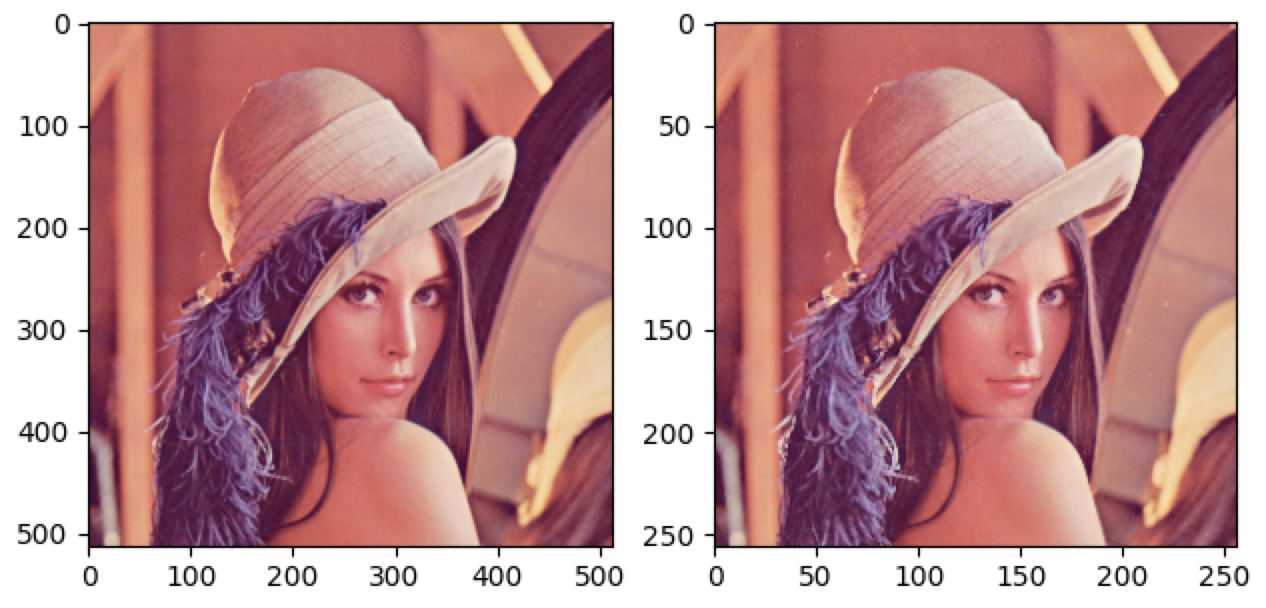#### nn.AvgPool2d

1
2
3
4
5
6
7
8
nn.AvgPool2d(
kernel_size,
stride=None,
ceil_mode=False,
divisor_override=None
)


• kernel_size：池化核尺寸。
• stride：步长。
• padding：填充个数。
• ceil_mode：尺寸向上取整。
• count_include_pad：是否将填充值用于平均值的计算。
• divisor_override：除法因子。计算平均值时代替像素个数作为分母。

1
2
3
4
5
6
7
8
9
10
11
12
13
14
15
16
17
18
19
20
21
22
23
24
25
26
27
28
29
30
import os
import torch
import torch.nn as nn
from torchvision import transforms
from matplotlib import pyplot as plt
from PIL import Image
from tools.common_tools import transform_invert, set_seed

set_seed(1)  # 设置随机种子

path_img = os.path.join(os.path.dirname(os.path.abspath(__file__)), "lena.png")
img = Image.open(path_img).convert('RGB')  # 0~255

# convert to tensor
img_transform = transforms.Compose([transforms.ToTensor()])
img_tensor = img_transform(img)
img_tensor.unsqueeze_(dim=0)    # C*H*W to B*C*H*W

# ========================== create avgpool layer =============================
avgpoollayer = nn.AvgPool2d((2, 2), stride=(2, 2))   # input:(i, o, size) weights:(o, i , h, w)
img_pool = avgpoollayer(img_tensor)

# =============================== visualization ===============================
print("池化前尺寸:{}\n池化后尺寸:{}".format(img_tensor.shape, img_pool.shape))
img_pool = transform_invert(img_pool[0, 0:3, ...], img_transform)
img_raw = transform_invert(img_tensor.squeeze(), img_transform)
plt.subplot(122).imshow(img_pool)
plt.subplot(121).imshow(img_raw)
plt.show()


1
2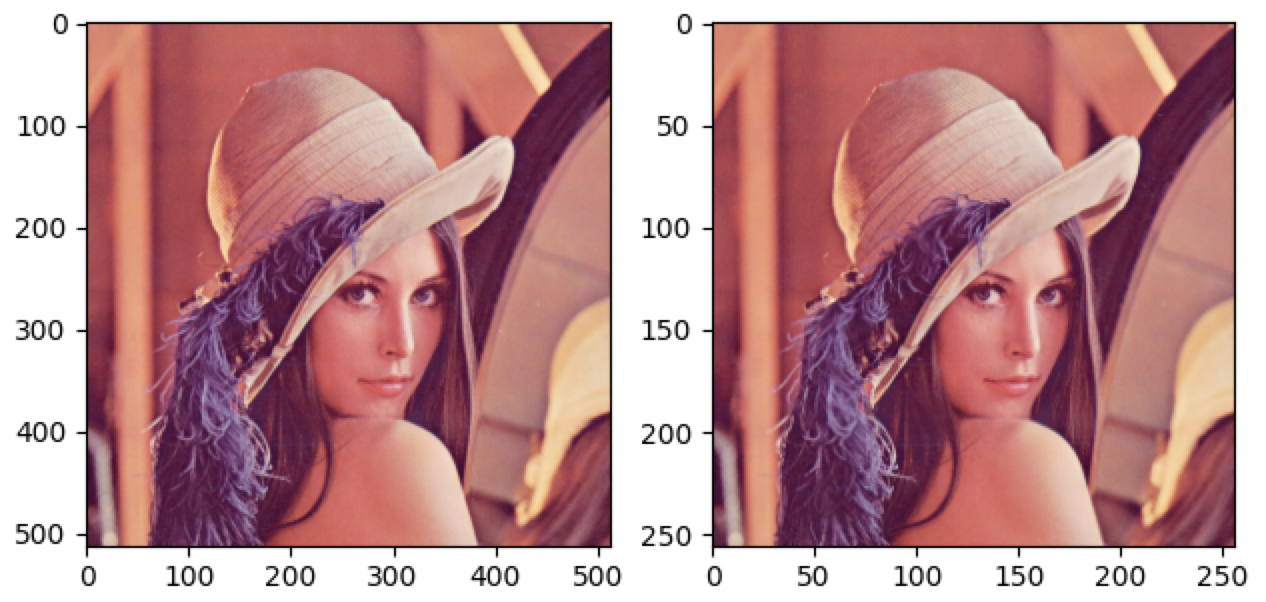##### divisor_override 的使用

1
2
3
4
5
img_tensor = torch.ones((1, 1, 4, 4))
avgpool_layer = nn.AvgPool2d((2, 2), stride=(2, 2))
img_pool = avgpool_layer(img_tensor)

print("raw_img:\n{}\npooling_img:\n{}".format(img_tensor, img_pool))


1
2
3
4
5
6
7
8
raw_img:
tensor([[[[1., 1., 1., 1.],
[1., 1., 1., 1.],
[1., 1., 1., 1.],
[1., 1., 1., 1.]]]])
pooling_img:
tensor([[[[1., 1.],
[1., 1.]]]])


$\dfrac{1+1+1+1}{4} = 1$

divisor_override=3 的平均池化

1
2
3
4
5
img_tensor = torch.ones((1, 1, 4, 4))
avgpool_layer = nn.AvgPool2d((2, 2), stride=(2, 2), divisor_override=3)
img_pool = avgpool_layer(img_tensor)

print("raw_img:\n{}\npooling_img:\n{}".format(img_tensor, img_pool))


1
2
3
4
5
6
7
8
raw_img:
tensor([[[[1., 1., 1., 1.],
[1., 1., 1., 1.],
[1., 1., 1., 1.],
[1., 1., 1., 1.]]]])
pooling_img:
tensor([[[[1.3333, 1.3333],
[1.3333, 1.3333]]]])


$\dfrac{1+1+1+1}{3} = 1.3333$

#### nn.MaxUnpool2d

1
2
3
4
5
6
7
8
nn.MaxUnpool2d(
kernel_size,
stride=None,
)

forward(self, input, indices, output_size=None)


• kernel_size：池化核尺寸。
• stride：步长。
• padding：填充个数。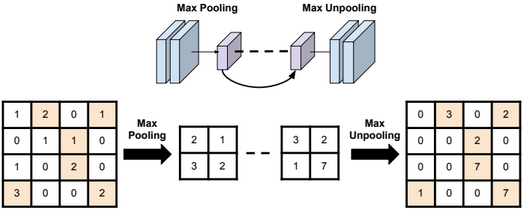1
2
3
4
5
6
7
8
9
10
11
12
# pooling
img_tensor = torch.randint(high=5, size=(1, 1, 4, 4), dtype=torch.float)
maxpool_layer = nn.MaxPool2d((2, 2), stride=(2, 2), return_indices=True)
img_pool, indices = maxpool_layer(img_tensor)

# unpooling
img_reconstruct = torch.randn_like(img_pool, dtype=torch.float)
maxunpool_layer = nn.MaxUnpool2d((2, 2), stride=(2, 2))
img_unpool = maxunpool_layer(img_reconstruct, indices)

print("raw_img:\n{}\nimg_pool:\n{}".format(img_tensor, img_pool))
print("img_reconstruct:\n{}\nimg_unpool:\n{}".format(img_reconstruct, img_unpool))


1
2
3
4
5
6
7
8
9
10
11
12
13
14
15
16
raw_img:
tensor([[[[0., 4., 4., 3.],
[3., 3., 1., 1.],
[4., 2., 3., 4.],
[1., 3., 3., 0.]]]])
img_pool:
tensor([[[[4., 4.],
[4., 4.]]]])
img_reconstruct:
tensor([[[[-1.0276, -0.5631],
[-0.8923, -0.0583]]]])
img_unpool:
tensor([[[[ 0.0000, -1.0276, -0.5631,  0.0000],
[ 0.0000,  0.0000,  0.0000,  0.0000],
[-0.8923,  0.0000,  0.0000, -0.0583],
[ 0.0000,  0.0000,  0.0000,  0.0000]]]])


## 2. 线性层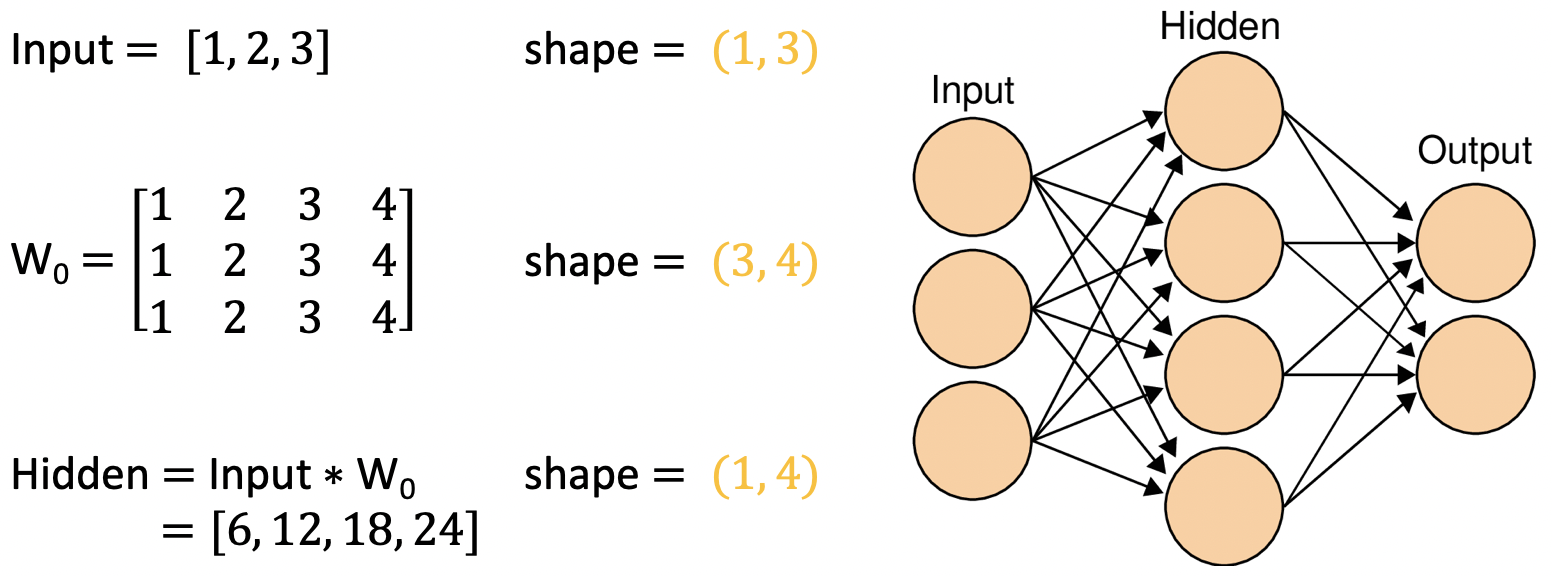#### nn.Linear

1
nn.Linear(in_features, out_features, bias=True)


• in_features：输入结点数。
• out_features：输出结点数。
• bias：是否需要偏置。

$y = x W^{\mathrm T} + \text{bias}$

1
2
3
4
5
6
7
8
9
10
11
12
inputs = torch.tensor([[1., 2, 3]])
linear_layer = nn.Linear(3, 4)
linear_layer.weight.data = torch.tensor([[1., 1., 1.],
[2., 2., 2.],
[3., 3., 3.],
[4., 4., 4.]])
linear_layer.bias.data.fill_(0.5)
output = linear_layer(inputs)

print(inputs, inputs.shape)
print(linear_layer.weight.data, linear_layer.weight.data.shape)
print(output, output.shape)


1
2
3
4
5
6
tensor([[1., 2., 3.]]) torch.Size([1, 3])
tensor([[1., 1., 1.],
[2., 2., 2.],
[3., 3., 3.],
[4., 4., 4.]]) torch.Size([4, 3])


## 3. 激活函数层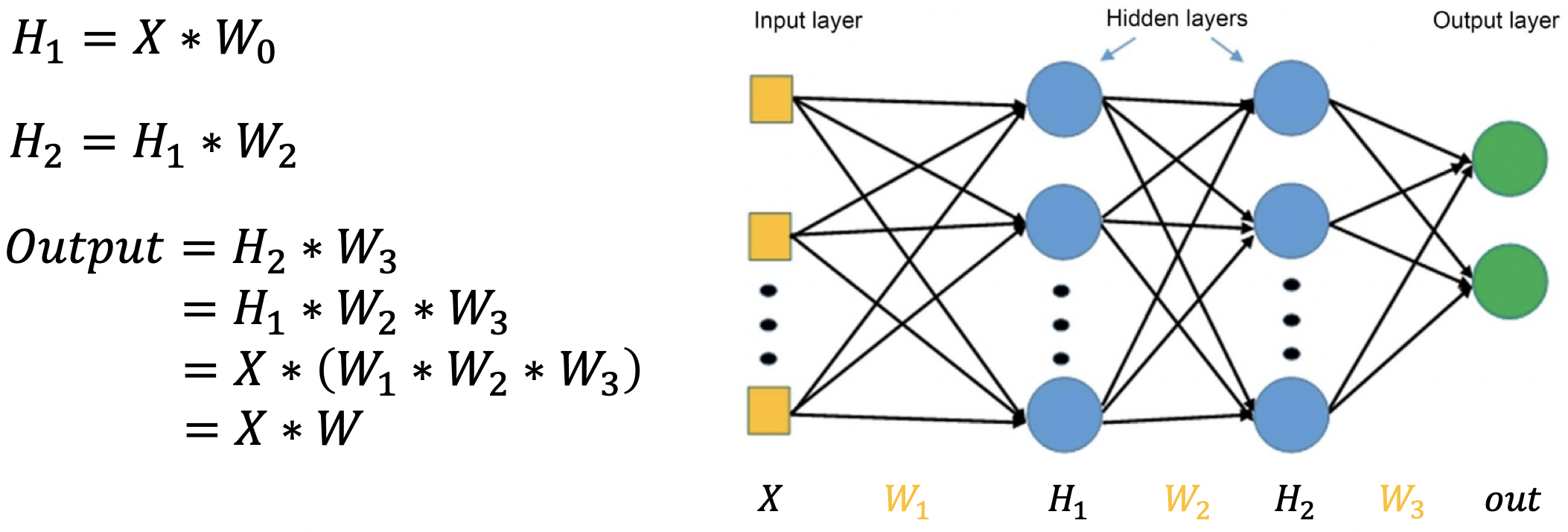#### nn.Sigmoid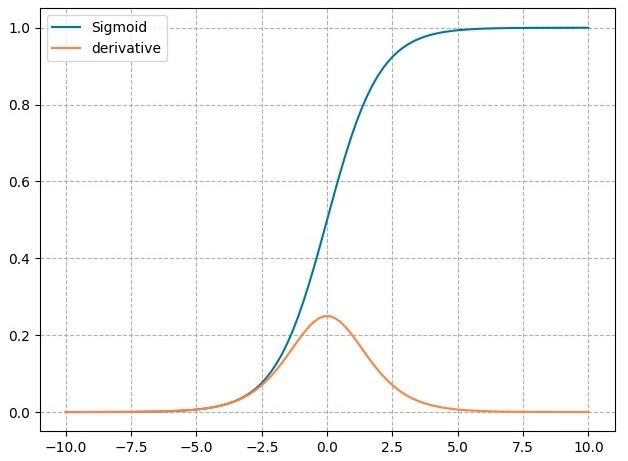$y = \dfrac{1}{1+e^{-x}}$

$y' = y*(1-y)$

• 输出值在 $(0,1)$，符合概率性质。
• 导数范围是 $[0, 0.25]$，容易导致梯度消失。
• 输出为非 $0$ 均值，会破坏数据分布。

#### nn.tanh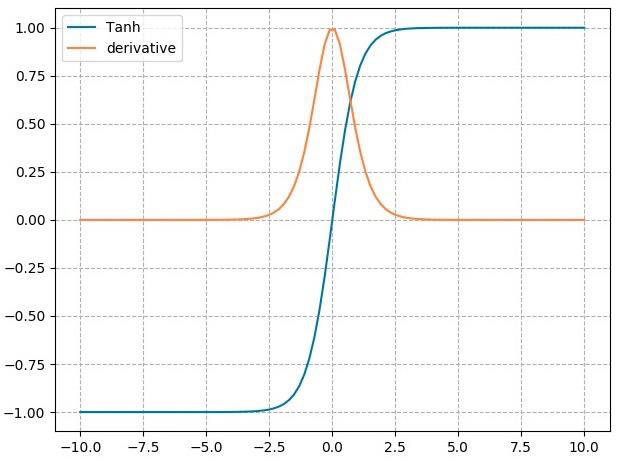$y= \dfrac{\sin x}{\cos x}=\dfrac{e^x - e^{-x}}{e^x + e^{-x}} = \dfrac{2}{1+e^{-2x}}+1$

$y' = 1- y^2$

• 输出值在 $(- 1,1)$，数据符合 $0$ 均值。
• 导数范围是 $(0, 1)$，容易导致梯度消失。

#### nn.ReLU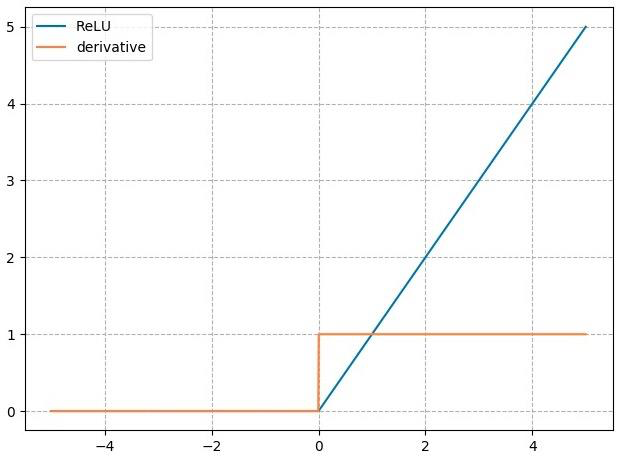$y= \max(0,x)$

$y'=\begin{cases}1\,, & x > 0 \\[2ex] \text{undefined}\,, & x=0 \\[2ex]0\,, & x < 0\end{cases}$

• 输出值均为正数，负半轴导致死神经元。
• 导数是 $1$，可以缓解梯度消失，但容易引发梯度爆炸。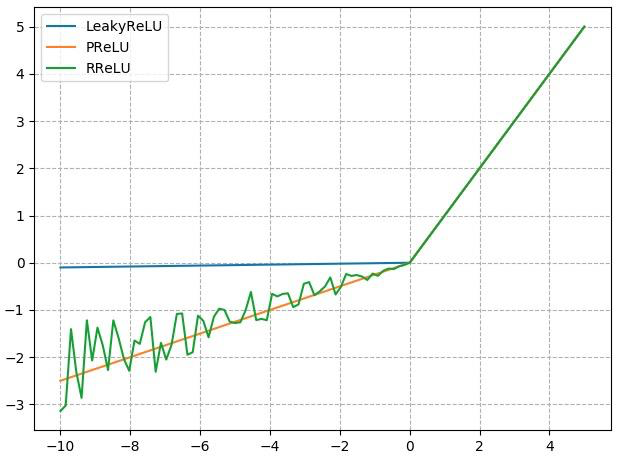#### nn.LeakyReLU

• negative_slope：负半轴斜率。

#### nn.PReLU

• init：可学习斜率。

#### nn.RReLU

• lower：均匀分布下限。
• upper：均匀分布上限。

## 4. 总结本作品采用知识共享署名-非商业性使用-相同方式共享 4.0 国际许可协议进行许可。 欢迎转载，并请注明来自：YEY 的博客 同时保持文章内容的完整和以上声明信息！